# Negative Feedback OpAmp Circuits

• #### Question 1

 Don’t just sit there! Build something!!

Learning to mathematically analyze circuits requires much study and practice. Typically, students practice by working through lots of sample problems and checking their answers against those provided by the textbook or the instructor. While this is good, there is a much better way.

You will learn much more by actually building and analyzing real circuits, letting your test equipment provide the “answers” instead of a book or another person. For successful circuit-building exercises, follow these steps:

1. Carefully measure and record all component values prior to circuit construction.
2. Draw the schematic diagram for the circuit to be analyzed.
3. Carefully build this circuit on a breadboard or other convenient medium.
4. Check the accuracy of the circuit’s construction, following each wire to each connection point, and verifying these elements one-by-one on the diagram.
5. Mathematically analyze the circuit, solving for all voltage and current values.
6. Carefully measure all voltages and currents, to verify the accuracy of your analysis.
7. If there are any substantial errors (greater than a few percent), carefully check your circuit’s construction against the diagram, then carefully re-calculate the values and re-measure.

Avoid using the model 741 op-amp, unless you want to challenge your circuit design skills. There are more versatile op-amp models commonly available for the beginner. I recommend the LM324 for DC and low-frequency AC circuits, and the TL082 for AC projects involving audio or higher frequencies.

As usual, avoid very high and very low resistor values, to avoid measurement errors caused by meter “loading”. I recommend resistor values between 1 kΩ and 100 kΩ.

One way you can save time and reduce the possibility of error is to begin with a very simple circuit and incrementally add components to increase its complexity after each analysis, rather than building a whole new circuit for each practice problem. Another time-saving technique is to re-use the same components in a variety of different circuit configurations. This way, you won’t have to measure any component’s value more than once.

• #### Question 2

The voltage gain of a single-ended amplifier is defined as the ratio of output voltage to input voltage: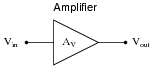AV = Vout Vin

Often voltage gain is defined more specifically as the ratio of output voltage change to input voltage change. This is generally known as the AC voltage gain of an amplifier:

 AV(AC) = ∆Vout ∆Vin

In either case, though, gain is a ratio of a single output voltage to a single input voltage.

How then do we generally define the voltage gain of a differential amplifier, where there are two inputs, not just one?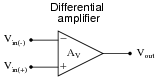• #### Question 3

Write the transfer function (input/output equation) for an operational amplifier with an open-loop voltage gain of 100,000. In other words, write an equation describing the output voltage of this op-amp (Vout) for any combination of input voltages (Vin(+) and Vin(−)):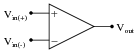• #### Question 4

How much voltage would have to be “dialed up” at the potentiometer in order to stabilize the output at exactly 0 volts, assuming the opamp has no input offset voltage?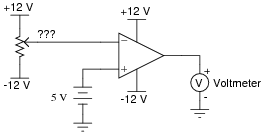• #### Question 5

An op-amp has 3 volts applied to the inverting input and 3.002 volts applied to the non-inverting input. Its open-loop voltage gain is 220,000. Calculate the output voltage as predicted by the following formula:

 Vout = AV ( Vin(+) − Vin(−) )

How much differential voltage (input) is necessary to drive the output of the op-amp to a voltage of -4.5 volts?

• #### Question 6

A very important concept in electronics is that of negative feedback. This is an extremely important concept to grasp, as a great many electronic systems exploit this principle for their operation and cannot be properly understood without a comprehension of it.

However important negative feedback might be, it is not the easiest concept to understand. In fact, it is quite a conceptual leap for some. The following is a list of examples - some electronic, some not - exhibiting negative feedback:

A voltage regulating circuit
An auto-pilot system for an aircraft or boat
A thermostatic temperature control system (“thermostat”)
Emitter resistor in a BJT amplifier circuit
Lenz’s Law demonstration (magnetic damping of a moving object)
Body temperature of a mammal
Natural regulation of prices in a free market economy (Adam Smith’s “invisible hand”)
A scientist learning about the behavior of a natural system through experimentation.

For each case, answer the following questions:

What variable is being stabilized by negative feedback?
How is the feedback taking place (step by step)?
What would the system’s response be like if negative feedback were not present?
• #### Question 7

Write the transfer function (input/output equation) for an operational amplifier with an open-loop voltage gain of 100,000, and the inverting input connected directly to its output terminal. In other words, write an equation describing the output voltage of this op-amp (Vout) for any given input voltage at the non-inverting input (Vin(+)):Then, once you have an equation written, solve for the over-all voltage gain $$(A_v = \frac{V_{out}}{V_{in(+)}})$$ of this amplifier circuit, and calculate the output voltage for a non-inverting input voltage of 6 volts.

• #### Question 8

How much effect will a change in the op-amp’s open-loop voltage gain have on the overall voltage gain of a negative-feedback circuit such as this?If the open-loop gain of this operational amplifier were to change from 100,000 to 200,000, for example, how big of an effect would it have on the voltage gain as measured from the non-inverting input to the output?

• #### Question 9

Complete the table of voltages for this opamp “voltage follower” circuit:Vin Vout 0 volts 0 volts 5 volts 10 volts 15 volts 20 volts -5 volts -10 volts -15 volts -20 volts

• #### Question 10

This operational amplifier circuit is often referred to as a voltage buffer, because it has unity gain (0 dB) and therefore simply reproduces, or “buffers,” the input voltage: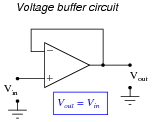What possible use is a circuit such as this, which offers no voltage gain or any other form of signal modification? Wouldn’t a straight piece of wire do the same thing? Explain your answers.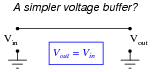• #### Question 11

For all practical purposes, how much voltage exists between the inverting and non-inverting input terminals of an op-amp in a functioning negative-feedback circuit?

• #### Question 12

Just as certain assumptions are often made for bipolar transistors in order to simplify their analysis in circuits (an ideal BJT has negligible base current, IC = IE, constant β, etc.), we often make assumptions about operational amplifiers so we may more easily analyze their behavior in closed-loop circuits. Identify some of these ideal opamp assumptions as they relate to the following parameters:

Magnitude of input terminal currents:
Input impedance:
Output impedance:
Input voltage range:
Output voltage range:
Differential voltage (between input terminals) with negative feedback:
• #### Question 13

The purpose of this circuit is to provide a pushbutton-adjustable voltage. Pressing one button causes the output voltage to increase, while pressing the other button causes the output voltage to decrease. When neither button is pressed, the voltage remains stable: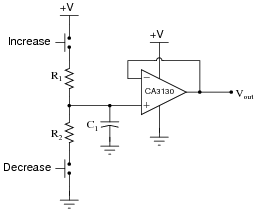After working just fine for quite a long while, the circuit suddenly fails: now it only outputs zero volts DC all the time.

An experienced technician first checks the power supply voltage to see if it is within normal limits, and it is. Then, the technician checks the voltage across the capacitor. Explain why this is a good test point to check, and what the results of that check would tell the technician about the nature of the fault.

• #### Question 14

A student builds the following regulated AC-DC power supply circuit, but is dissatisfied with its performance:The voltage regulation is not as good as the student hoped. When loaded, the output voltage “sags” more than the student wants. When the zener diode’s voltage is measured under the same conditions (unloaded output, versus loaded output), its voltage is noted to sag a bit as well. The student realizes that part of the problem here is loading of the zener diode through the transistor. In an effort to improve the voltage regulation of this circuit, the student inserts an opamp “voltage follower” circuit between the zener diode and the transistor: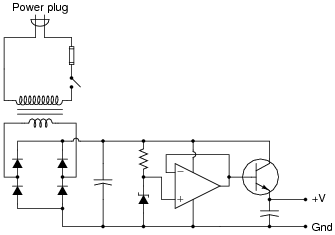Now the zener diode is effectively isolated from the loading effects of the transistor, and by extension from the output load as well. The opamp simply takes the zener’s voltage and reproduces it at the transistor base, delivering as much current to the transistor as necessary without imposing any additional load on the zener diode.

This modification does indeed improve the circuit’s ability to hold a steady output voltage under changing load conditions, but there is still room for improvement. Another student looks at the modified circuit, and suggests one small change that dramatically improves the voltage regulation: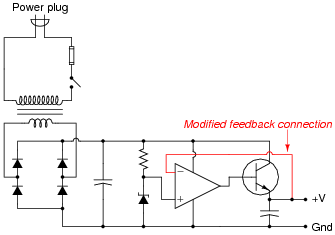Now the output voltage holds steady at the zener diode’s voltage with almost no “sag” under load! The second student is pleased with the success, but the first student does not understand why this version of the circuit functions any better than previous version. How would you explain this circuit’s improved performance to the first student? How is an understanding of negative feedback essential to being able to comprehend the operation of this circuit?

• #### Question 15

Predict how the operation of this regulated power supply circuit will be affected as a result of the following faults. Consider each fault independently (i.e. one at a time, no multiple faults):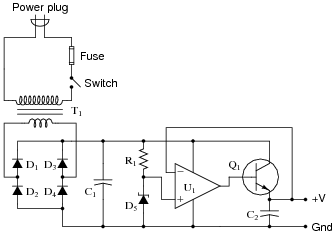Transformer T1 primary winding fails open:
Rectifying diode D3 fails open:
Rectifying diode D4 fails shorted:
Resistor R1 fails open:
Zener diode D5 fails open:
Operational amplifier U1 fails with output saturated positive:
Transistor Q1 fails open (collector-to-emitter):

For each of these conditions, explain why the resulting effects will occur.

• #### Question 16

This regulated power supply circuit has a problem. Instead of outputting 15 volts DC (exactly) as it should, it is outputting 0 volts DC to the load: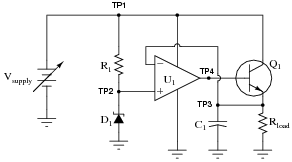You measure 0.25 volts DC between TP4 and ground, and 20 volts between TP1 and ground, using your voltmeter. From this information, determine at least two independent faults that could cause this particular problem.

• #### Question 17

“Split” or “dual” DC power supplies are essential for powering many types of electronic circuits, especially certain types of operational amplifier circuits. If only a ßingle” DC power supply is not available, a ßplit” power supply may be roughly simulated through the use of a resistive voltage divider: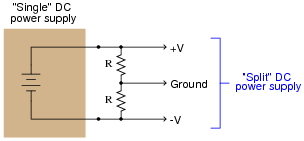The problem with doing this is loading: if more current is drawn from one of the power supply rails than from the other, the “split” of voltage will become uneven. The only way that V and -V will have the same (absolute) voltage value at the load is if the load impedance is balanced evenly between those rails and ground. This scenario is unlikely. Take for instance this example: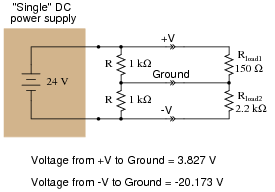A simple opamp circuit, though, can correct this problem and maintain an even “split” of voltage between V, Ground, and -V: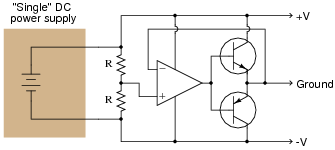Explain how this circuit works. What function do the two resistors perform? How is negative feedback being used in this circuit?

• #### Question 18

Design a passive circuit that will create a “split” ( V/-V) power supply from a single voltage source:• #### Question 19

A complementary push-pull transistor amplifier built exactly as shown would perform rather poorly, exhibiting crossover distortion: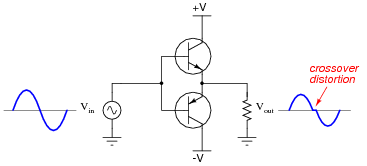The simplest way to reduce or eliminate this distortion is by adding some bias voltage to each of the transistors’ inputs, so there will never be a period of time when the two transistors are simultaneously cutoff: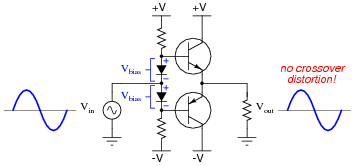One problem with this solution is that just a little too much bias voltage will result in overheating of the transistors, as they simultaneously conduct current near the zero-crossing point of the AC signal. A more sophisticated method of mitigating crossover distortion is to use an opamp with negative feedback, like this: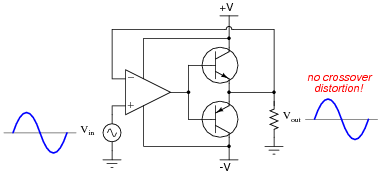Explain how the opamp is able to eliminate crossover distortion in this push-pull amplifier circuit without the need for biasing.

• #### Question 20

The parasitic capacitance naturally existing in two-wire cables can cause problems when connected to high-impedance electronic devices. Take for instance certain biomedical probes used to detect electrochemical events in living tissue. Such probes may be modeled as voltage sources in series with resistances, those resistances usually being rather large due to the probes’ very small surface (contact) areas: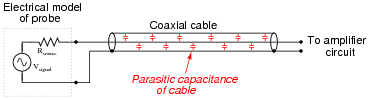When connected to a cable with parasitic capacitance, a low-pass RC filter circuit is formed: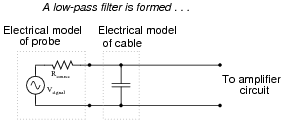This low-pass filter (or passive integrator, if you wish) is purely unintentional. No one asked for it to be there, but it is there anyway just due to the natural resistance of the probe and the natural capacitance of the cable. Ideally, of course, we would like to be able to send the signal voltage (Vsignal) straight to the amplifier with no interference or filtering of any kind so we can see exactly what it is we’re trying to measure.

One clever way of practically eliminating the effects of cable capacitance is to encase the signal wire in its own shield, and then drive that shield with the exact same amount of voltage from a voltage follower at the other end of the cable. This is called guarding:An equivalent schematic may make this technique more understandable: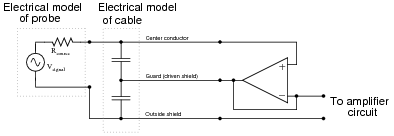Explain why guarding the signal wire effectively eliminates the effects of the cable’s capacitance. Certainly the capacitance is still present, so how can it not have any effect on the weak signal any more?

• #### Question 21

Ideally, the input terminals of an operational amplifier conduct zero current, allowing us to simplify the analysis of many opamp circuits. However, in actuality there is a very small amount of current going through each of the input terminals of any opamp with BJT input circuitry. This may cause unexpected voltage errors in circuits.

Consider the following voltage buffer circuit: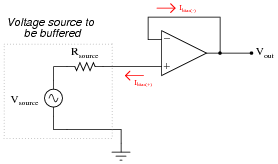Ibias(−) does not cause any trouble for us, because it is completely supplied by the opamp’s output. The other bias current, though, does cause trouble, because it must go through the source’s Thévenin resistance. When it does, it drops some voltage across that intrinsic source resistance, skewing the amount of voltage actually seen at the non-inverting terminal of the opamp.

A common solution to this is to add another resistor to the circuit, like this: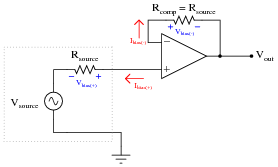Explain why the addition of a resistor fixes the problem.